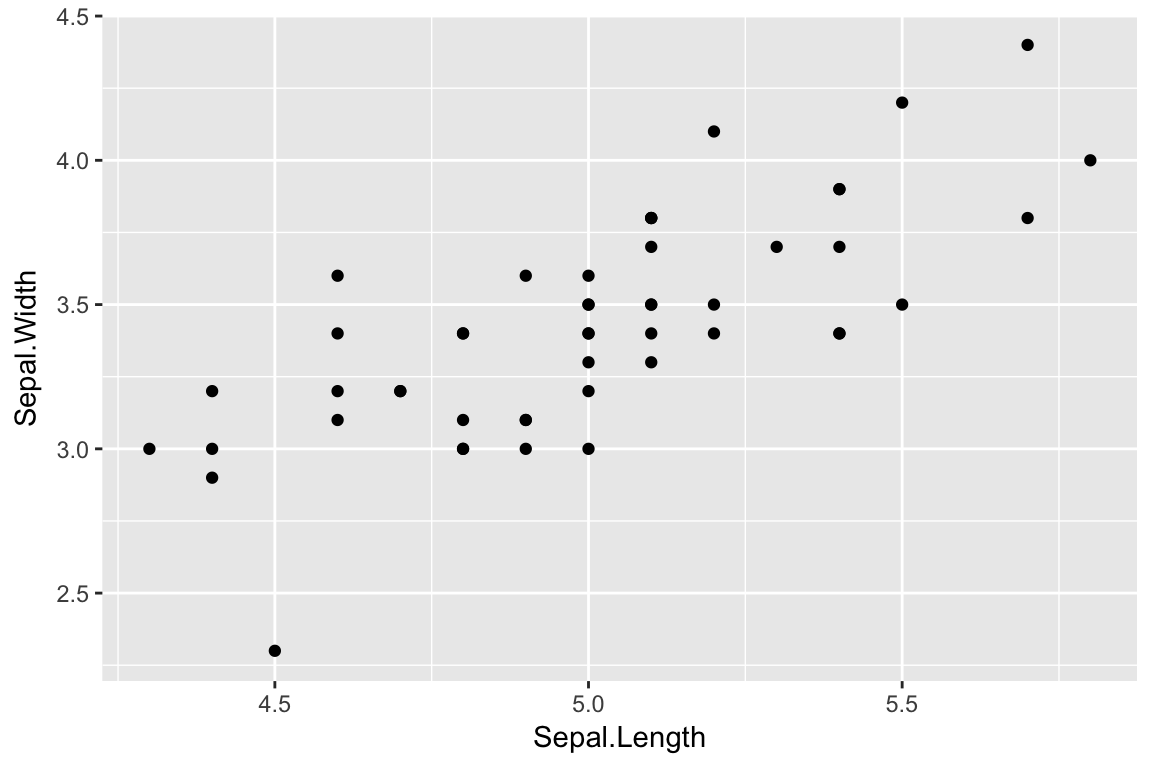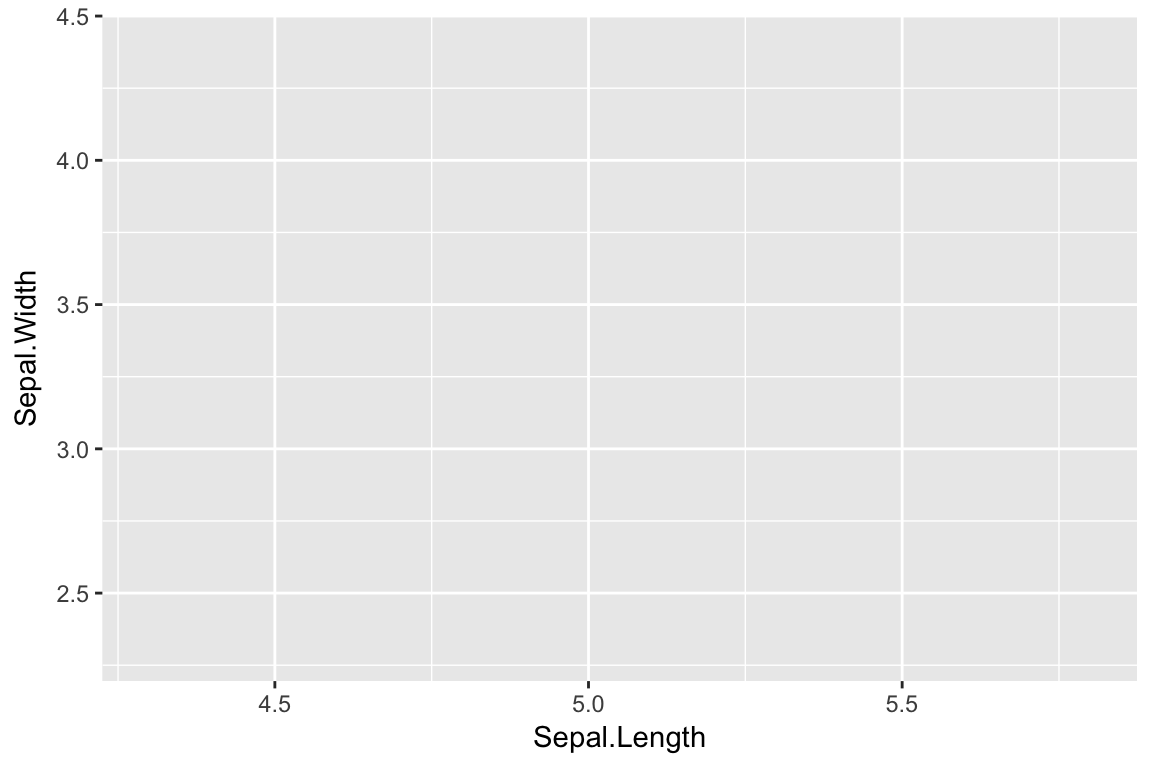# Introduction

Having already explored various ways to visualize the distributions of single variables (or one continuous variable plus a qualitative variable), we now turn our attention to bivariate and multivariate representations.

``library(tidyverse)``

# Subsetting data using `dplyr::filter`

We’ll continue to use the `iris` data set for our examples.

Initially we’ll use just the I. setosa specimens from the Iris data set for our visualizations. To select just the I. setosa specimens, we introduce a function called `filter` which is found in the `dplyr` package (part of the tidyverse!).

CAUTION: there is a built in `filter` function as well, so make sure you load tidyverse or dplyr to get the expected behavior.

`filter` takes the rows of a data frame that match the specified criteria. For example, the following returns only the setosa specimens:

``````setosa <- filter(iris, Species == "setosa")
dim(setosa)``````

If we wanted to get both setosa and virginica specimens we could use the OR operator (`|`) as so:

``````setosa.or.virginica <- filter(iris, Species == "setosa" | Species == "virgnica")
dim(setosa.or.virginica)``````

You can also create AND statement using the `&` operator. For example, if you wanted to get only setosa specimens with sepal widths bigger than 3.5 cm you could do:

``````big.setosa <- filter(iris, Species == "setosa" & Sepal.Width > 3.5)
dim(big.setosa)``````

# In-class Assignment #1

Create a R Markdown notebook, load any necessary libraries, and read the `possums.csv` data set. Write code blocks to solve the following problems:

1. Use dply `filter` function to create a new data frame with just the female possums [1 pt]

2. Use `filter` to create a new data frame that includes only female possums that were assigned to age class 9. How many possums meet these criteria? [1 pt]

3. Use `filter` to select those possums in age class 5 or older, or for which age information is unavailable (hint: see the help for the `is.na` function ). How many possums meet these criteria? [2 pts]

# Scatter plots

A scatter plot is one of the simplest representations of a bivariate distribution. Scatter plots are simple to create in ggplot2 by specifying the appropriate X and Y variables in the aesthetic mapping and using `geom_point` for the geometric mapping.

``````ggplot(setosa)  +
geom_point(aes(x = Sepal.Length, y = Sepal.Width))``````# gplot layers can be assigned to variables

`ggplot` not only facilitates drawing of plots, but also returns a “plot object” that we can assign to a variable. The following example illustrates this:

``````# create base plot object and assign to variable p
# this does NOT draw the plot
p <- ggplot(setosa, mapping = aes(x = Sepal.Length, y = Sepal.Width))   ``````

In the code above we created a plot object and assigned it to the variable `p`. However, the plot wasn’t drawn. To draw the plot object we evaluate it as so:

``p  # draw the plot object``Did the output from this code generate the image you expected?

In the previous steps we created a plot object, and drew it, but we haven’t yet specified a geom to determine how our data should be drawn. As we’ve seen in the past, geoms are layers that we can add to our plot object. Here we simply add a geom to our pre-created plot object as so:

``````# add a point geom to our base layer and draw the  plot
p + geom_point()``````